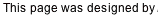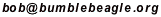## Du-Sum-Oh Solving Tutorial

Below is the puzzle we want to solve. We need to place the letters A-G in the cells so that each row contains exactly one of each letter. Ditto for each column and for each region. Several letters have been given to start us off.

This tutorial refers to rows and columns by number, and to regions by the starter letter in the region.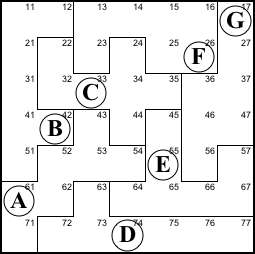Step 1. Column 1 and region B share the five purple cells below left. Thus the two pink cells that complete column 1 and the two blue cells that complete region B must contain the same two symbols. So cell 12 must be A and cell 71 must be B. The result is shown below right.

12=A, 71=B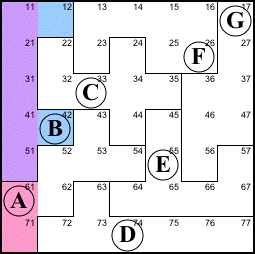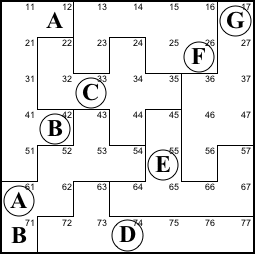Step 2. Similarly, row 7 and region D share the six purple cells below, so the pink cell (which completes region D) must be the same as the blue cell (which completes row 7).

63=B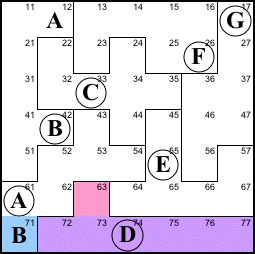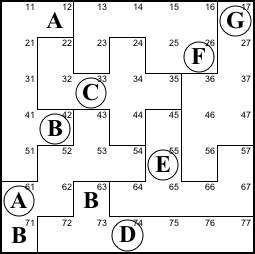Step 3. Row 1 and region F and share the four purple cells, so the three pink cells must contain the same symbols as the three blue cells. The pink cells already contain A and G, so they must pick up the F from 26 to fill 11. 23 and 25 will eventually be A and G but we can't yet determine which is which.

11=F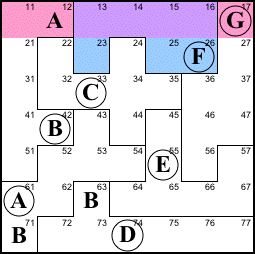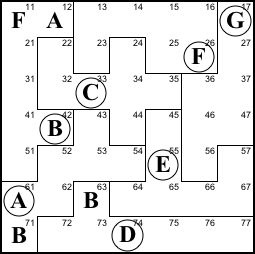Step 4. Comparing row 6 and region E we see that the three blue cells must be the same as the three pink cells. They must contain A, B, and E, so the pink cells need E in 62. The blue cells need an A and a B, but row 5 already has B, so 45 is A. That leaves B for 57.

This can be described as the little law of leftovers. Wherever a region overlaps a row or column, the parts outside the overlap (the leftovers) have to be the same.

E=62, 45=A, 57=B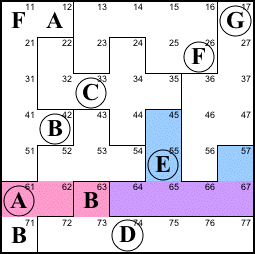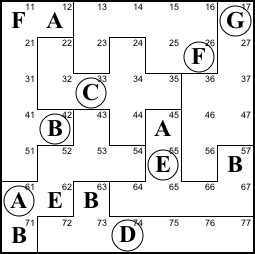Step 5. Row 5 and region A reveal that the pink cells must contain A, B, E, and something else. Column 1 has an A so 51 can't be A. The A must be at 56.

Even though we don't yet know what the "something else" is, we know that cells 43 and 51 are the same. This knowledge could help us (though in this puzzle it isn't needed).

56=A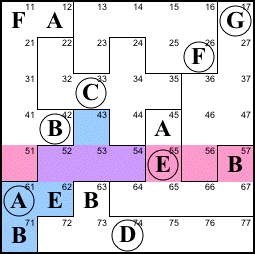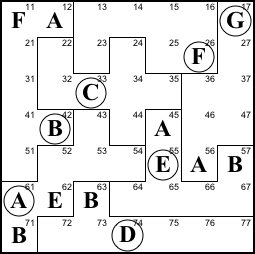Step 6. Column 7 and region G reveal that the pink cells need B and the blue cells need A. 46 can't be B (due to 42) so 36 is B. And 67 can't be A (due to 61) so 77 is A.

36=B, 77=A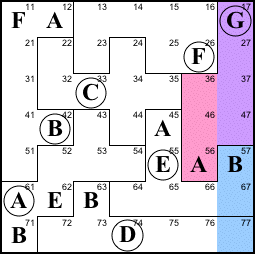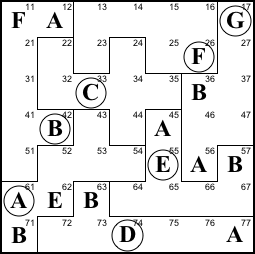Step 7. We have now assigned five As; there are only two left. They have to be in rows 2 and 3 and columns 3 and 4, the purple cells. The only possibility that keeps them in a different row and column is 23 and 34. And looking again at row 1/region F (as we did in step 3), we see that 25 is G.

23=A, 34=A, 25=G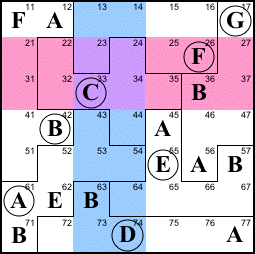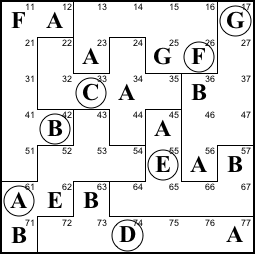Step 8. We have also assigned five Bs; the two left must be in the purple cells, so they must be 14 and 15.

24=A, 15=A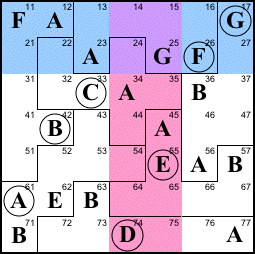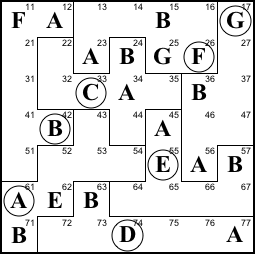Step 9. Consider region C. It lacks D, E, F, and G. Columns 2 and 5 contain E, the 44 is E. The G at 25 kills the possibility of G at 22 and 35, so 32 is G. The F in row 2 forces the F to 35. And the only place left for the D is 22.

44=E, 32=G, 35=F, 22=D.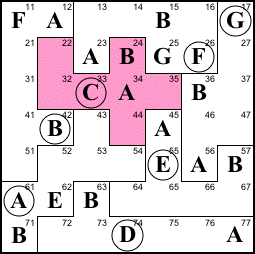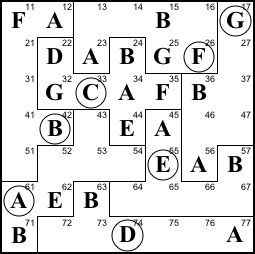Step 10. Column 5 lacks C and D. The D at 74 forces 75 to C and 65 to D.

75=C, 65=D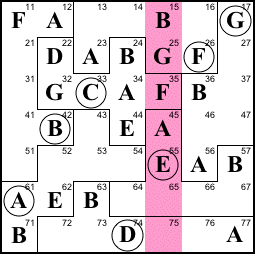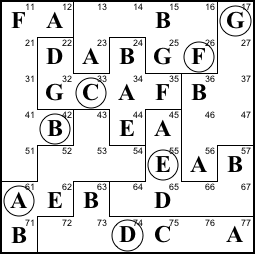Step 11. Similarly, column 2 lacks C and F, and the C at 75 forces 72 to F and 52 to C.

72=F, 52=C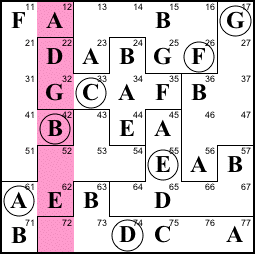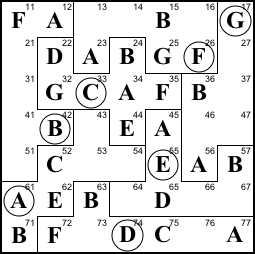Step 12. Row 1 lacks C, D, and E. Column 4 already contains D and E, so 14 is C. forces 72 to F and 52 to C.

14=C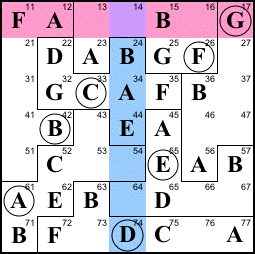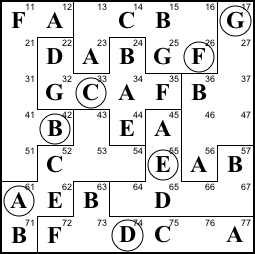Step 13. Rows 2 and 3 and column 6 contain F, so the only spot left for an F in region G is 47.

47=F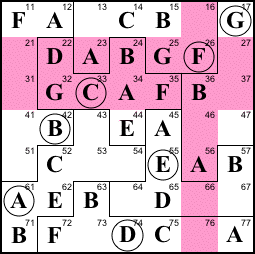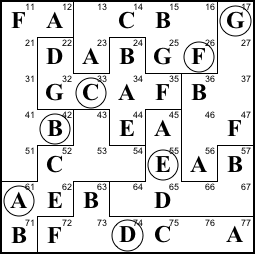Step 14. Row 6 lack C, F, and G. The Fs at 26 and 47 force F to 64. Then the G at 17 forces G to 66 and C to 67.

64=F, 66=G, 67=C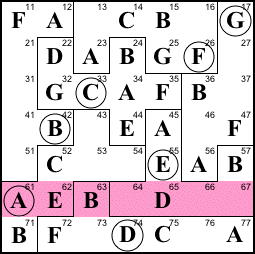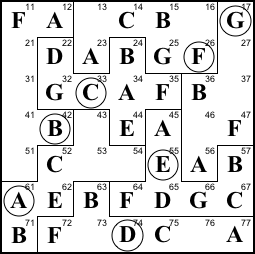Step 15. Column 7 gets D in 37 (due to D in 22) and E in 27. This allows us to finish off row 2 with C at 21, row 3 with E at 31, and region G with C at 46.

37=D, 27=E, 21=C, 31=E, 46=C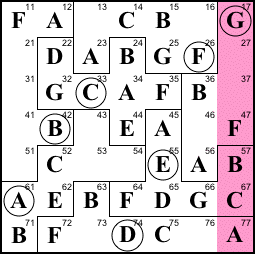Solution. At this point things easily fall in place.

54=G, 76=E, 16=D, 13=E, 43=D, cell 53=F, 73=G, 51=D, 41=G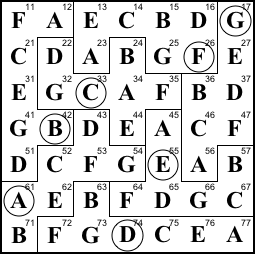Back to Bob’s main Du-Sum-Oh page.